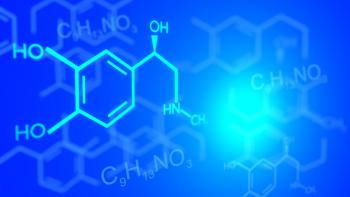# Solar Energy# Chemical ThermodynamicsChemical thermodynamics (or thermochemistry) is the branch of thermodynamics that studies the thermal effects caused by chemical reactions, called the heat of reaction. Therefore, chemical thermodynamics refers to the conversions of chemical energy into thermal energy and vice versa, which occur during a reaction and studies the variables connected to them, such as bond enthalpy, entropy of standard formation, etc. All these conversions are made within the limits of the laws of thermodynamics.

In addition to the thermodynamic principles there are two laws governing the entire discipline of thermochemistry:

• Lavoisier and Laplace Law (formulated in 1780): the heat transfer that accompanies a given chemical reaction is the same and contrary to the heat transfer of the opposite reaction;
• Hess's Law (formulated in 1840): the variation of the enthalpy of reaction is the same as the reaction occurs in one or more successive and independent stages (even purely hypothetical).

The two laws were empirically deduced and enunciated before the first principle of thermodynamics: however, you can prove that they are direct consequences of it, as well as the fact that enthalpy H and internal energy U are thermodynamic functions of the state.

Chemical thermodynamics involves not only laboratory measurements of various thermodynamic properties, but also the application of mathematical methods for the study of chemical questions and the spontaneity of processes.

In photovoltaic solar energy, chemical thermodynamics has special relevance in the charging and discharge of batteries. During this process an exchange between chemical energy and electricity is experienced.

## Description of chemical thermodynamics

The main objective of chemical thermodynamics is the establishment of a criterion to determine the feasibility or spontaneity of a given transformation. In this way, chemical thermodynamics is typically used to predict the energy exchanges that occur in the following processes: chemical reactions, phase changes, solution formation.

The following state functions are of primary interest in chemical thermodynamics: internal energy (U), enthalpy (H), entropy (S), Gibbs free energy (G).

The structure of chemical thermodynamics is based on the first two laws of thermodynamics. From the first law of thermodynamics and the second law of thermodynamics, four equations called "Gibbs fundamental equations" can be derived. From these four, a multitude of equations can be derived, which relate the thermodynamic properties of the thermodynamic system, using relatively simple mathematics. This delineates the mathematical framework of chemical thermodynamics.

## Thermodynamic systems

In thermochemistry there are several very useful thermodynamic definitions. A thermodynamic system is the specific portion of the universe that is being studied. Everything outside the system is considered environment or environment. A system can be:

• A thermodynamic (completely) insulated system that cannot exchange energy or matter with the environment, such as an isolated pump calorimeter.
• A thermally insulated system that can exchange mechanical work, but not heat or matter, such as an insulated closed piston or balloon.
• A mechanically insulated system that can exchange heat, but not work or mechanical matter, such as a non-insulated pump calorimeter
• A closed system that can exchange energy, but it doesn't matter, like a closed balloon or piston without insulation
• An open system that can exchange matter and energy with the environment, such as a pot of boiling water

## Chemical reactions

In most cases of interest in chemical thermodynamics, there are internal degrees of freedom and processes, such as chemical reactions and phase transitions. These chemical reactions always create entropy unless they are in equilibrium or are maintained in a continuous equilibrium.

Even for homogeneous materials, free energy functions depend on the composition, as do all extensive thermodynamic potentials, including internal energy.

## History of chemical thermodynamics

In 1865, the German physicist Rudolf Clausius, in his Mechanical Theory of Heat, suggested that the principles of thermochemistry, for example, the heat generated in combustion reactions, could be applied to the principles of thermodynamics. Based on the work of Clausius, between 1873-76 the American mathematical physicist Willard Gibbs published a series of three articles, the most famous was the document on the balance of heterogeneous substances.

In these articles, Gibbs showed how the first two laws of thermodynamics could be measured graphically and mathematically to determine both the thermodynamic equilibrium of chemical reactions and their tendencies to occur or move forward. Gibbs' collection of documents provided the first unified body of thermodynamic theorems from the principles developed by others, such as Clausius and Sadi Carnot.

At the beginning of the 20th century, two important publications successfully applied the principles developed by Gibbs to chemical processes, and thus established the foundations of the science of chemical thermodynamics. The first was the 1923 textbook Thermodynamics and Chemical Free Energy by Gilbert N. Lewis and Merle Randall. This book was responsible for supplanting chemical affinity with the term free energy in the English-speaking world.

The second was the 1933 book Modern Thermodynamics by the methods of Willard Gibbs, written by EA Guggenheim. Thus, Lewis, Randall and Guggenheim are considered the founders of modern chemical thermodynamics due to the great contribution of these two books to Unify the application of thermodynamics to chemistry.

valoración: 3 - votos 1

Last review: September 24, 2019